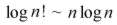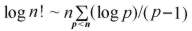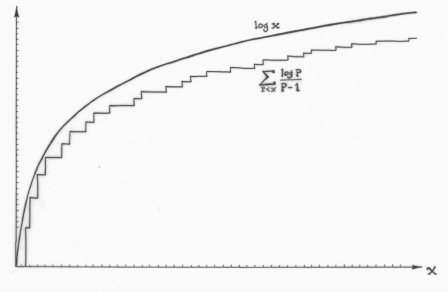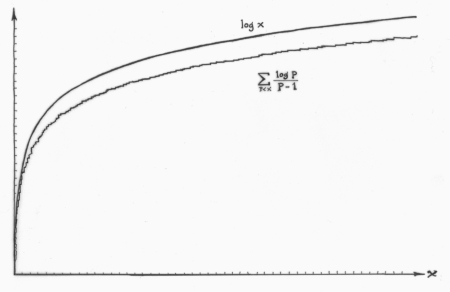### "The prime number theorem obtained by statistical methods"

from What is Mathematics? by R. Courant and H. Robbins

Near the end of the book, we find a section with this name. The authors state:

"By a procedure typical of...statistical mechanics we... [make] plausible the...law of the distribution of primes."

Two results are combined:which was proven earlier in the book, by means of a fairly straightforward argument, andwhich is proven in this section, using a simple counting argument,

to give an elementary result with an elementary proof:Note that the RHS is a simple step function based on the positions of the primes. The above result states that it is asymptotic to the natural logarithm function.

Here we see the two functions in the range [0,100]:Here we see the two functions in the range [0,1000]:This relationship between the logarithm function and the distribution of primes is possibly the best "suggestive" evidence for the prime number theoremThe authors assume the existence of a density function w(x) for the primes, with certain basic properties, and then rewritein terms of an integral involving this density. Elementary calculus methods are then used to deduce that

w(x) ~ (x - 1)/xlog x,

leading to the prime number theorem.

number theory and physics archive
tutorial      mystery      new      search      home